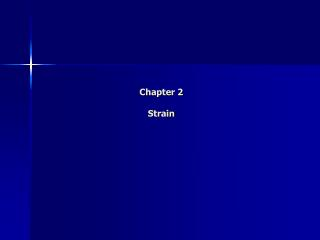DownloadDownload PresentationChapter 2 Strain

# Chapter 2 Strain

Télécharger la présentation## Chapter 2 Strain

- - - - - - - - - - - - - - - - - - - - - - - - - - - E N D - - - - - - - - - - - - - - - - - - - - - - - - - - -
##### Presentation Transcript

1. Chapter 2Strain

2. Deformation • Whenever a force is applied to a body, it will undergo deformation (change the body's shape and size) • The deformation can be highly visible, but is often unnoticeable • Generally, the deformation of a body will not be uniform throughout its volume → so the change in geometry of any line segment within the body may vary along its length • To study deformational changes in a more uniform manner, lines will be considered that are very short and located in the vicinity of a point

3. Normal Strain • The elongation or contraction of a line segment per unit of length is referred to as normal strain (ε) • The average normal strain • The normal strain at point A in the direction of n • Approximate final length of a short line segment in the direction of n • Normal strain is a dimensionless quantity (but often still use units representing the ratio of length, mm/mm or in/in)

4. Shear Strain • The change in angle that occurs between two line segments that were originally perpendicular to one another is referred to as shear strain • The shear strain at point A that is associated with n and t axes • If θ' is smaller than π/2 the shear strain is positive, if θ' is larger than π/2 the shear strain is negative • Shear strain is measured in radians (rad)

5. Cartesian Strain Components • Normal strains cause a change in volume of the element, whereas the shear strains cause a change in its shape

6. Small Strain Analysis • Most engineering design involves applications for which only small deformations are allowed • It is assumed in this text that deformations occurring within a body are almost infinitesimal, so that normal strains are very small compared to 1 (ε<<1, referred to as small strain analysis) • If θ is very small, can approximate (in radians) sin θ = θ, tan θ = θ, cosθ = 1 • Problems pg 75